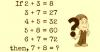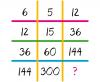BRAIN TEASERSBrain Teasers User Profile

 Solve Math Puzzle If 2 + 3 = 8, 3 + 7 = 27, 4 + 5 = 32, 5 + 8 = 60, 6 + 7 = 72, then, 7 + 8 = ?MATH PUZZLE: Can you replace... MATH PUZZLE: Can you replace the question mark with a number?